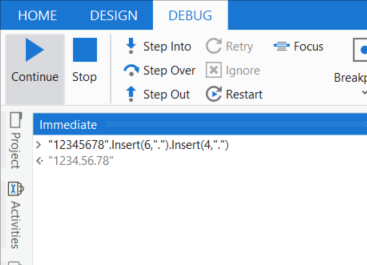# How put decimal on a number

I have a situation where I am fetching a 8 digit number from a portal and everytime I am storing it into a variable called num

Now I want to insert two decimal points in the number (specifically after the 4th digit and the 6th digit) and then write that number to a excel file,

For example if the number fetched from the portal is 12345678

In excel it should be written as 1234.56.78

How to achieve this ?

Please try the below expression provided if it is always eight digit

yourvariable.tostring.substring(0,4)+“.”+yourvariable.tostring.substring(4,2)+“.”+yourvariable.tostring.substring(6,2)

if it is not eight digit always you can use the below expression

yourvariable.tostring.substring(0,yourvariable.tostring.Length-4)+“.”+yourvariable.tostring.substring(yourvariable.tostring.Length-4,2)+“.”+yourvariable.tostring.substring(yourvariable.tostring.Length-2,2)

Hello,

You can divide your inital string with substring function
InitVar.Substring(0,1) → Part 1
InitVar.subString(4,2) → Part 2
InitVar.subString(6,2) → Part 3
And then concatenate it adding dot

Let’s assume we can rely on the length:`YourVar.Insert(6,".").Insert(4,".")`

This topic was automatically closed 3 days after the last reply. New replies are no longer allowed.